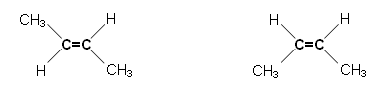# What is E-Z isomerism?

Jun 11, 2015

$E - Z$ isomerism is a type of stereoisomerism that exists because of restricted rotation about double bonds.

#### Explanation:

In stereoisomers, the atoms are joined in the same order, but they have a different spatial arrangement.

In $E - Z$ isomers you must have:

• restricted rotation, often involving a $\text{C=C}$ double bond
• two different groups on one end of the bond and two different groups on the other end.

An alkene such as but-2-ene has two different groups on each alkene carbon.

It can exist as $E - Z$ isomers that differ in the positions of the substituents on the double-bonded atoms.The substituents can be given "priorities", with atoms with higher atomic numbers given higher priorities (the Cahn-Ingold-Prelog rules).

If the highest priority groups for each carbon are on the same side of the molecule, we have the $Z$ isomer.

If the highest priority groups for each carbon are on opposite sides of the molecule, we have the $E$ isomer.One way to remember the designations is to think of $Z$ as Zame Zide (same side).

Since ${\text{CH}}_{3}$ has higher priority than $\text{H}$, it is pretty easy to determine that the butene isomers above are $E$ and $Z$, respectively.

But what about the isomers of 2-methylbut-2-enoic acid (below)?${\text{CH}}_{3}$ has higher priority than $\text{H}$, and $\text{COOH}$ has higher priority than ${\text{CH}}_{3}$.

Angelic acid has the higher priority groups on the same side, so it is the $Z$ isomer.

Tiglic acid has the higher priority groups on opposite sides, so it is the $E$ isomer.